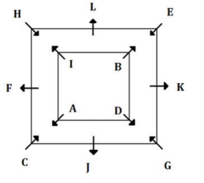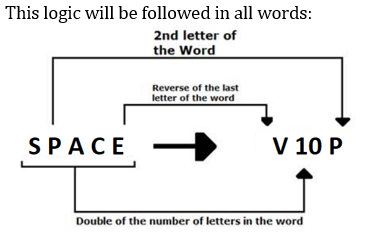Latest Banking jobs   »

# Reasoning Ability Quiz For SIDBI GRADE A 2022- 28th December

Directions (1-2): In these questions, relationship between different elements is shown in the statements. These statements are followed by two conclusions. Mark answer as

Q1. Statement: A≥M≥N=G>K, H>R=O≤N
Conclusion: I. A≥O
II. H>K
(a) If only conclusion II follows.
(b) If only conclusion I follows.
(c) If both conclusion I and conclusion II follow
(d) If neither conclusion I nor conclusion II follows.
(e) If either conclusion I or conclusion II follows.

Q2. Statement: H≥R≤K>E≤S, E=D≥G
Conclusion: I. G≥R
II. K>G
(a) If neither conclusion I nor conclusion II follows.
(b) If only conclusion I follows.
(c) If both conclusion I and conclusion II follow
(d) If only conclusion II follows.
(e) If either conclusion I or conclusion II follows.

Directions (3-5): In each question below is given a statement followed by two assumptions numbered I and II. An assumption is something supposed or taken for granted. You have to consider the statement and the following assumptions and decide which of the assumptions is/are implicit in the statement. Give answer:

Q3. Statement: The largest domestic airlines corporation has announced new summer schedules in which a greater number of flights in trunk routes are introduced.
Assumptions
I. More number of passengers may travel by this airlines corporation during summer months in trunk routes.
II. Other airlines companies may also increase the number of flights in all the sectors.
(a) if only Assumption I is implicit
(b) if only Assumption II is implicit
(c) if either Assumption I nor II is implicit
(d) if neither Assumption I nor II is implicit
(e) if both Assumption I and II are implicit

Q4. Statement: The chairman of the company decided to hold a grand function to celebrate silver jubilee during the next weekend and invited a large number of guests.
Assumptions
I. The company officials may be able to make all the necessary preparations for the silver jubilee celebration.
II. Majority of the guests invited by the chairman may attend the function.
(a) if only Assumption I is implicit
(b) if only Assumption II is implicit
(c) if either Assumption I nor II is implicit
(d) if neither Assumption I nor II is implicit
(e) if both Assumption I and II are implicit

Q5. Statements: The largest computer manufacturing company slashed the prices of most of the desktop models by about 15% with immediate effect.
Assumptions
I. The company may incur heavy losses due to reduction in prices of the desktop.
II. The sales of desktop manufactured by the company increases substantially in the near future.
(a) if only Assumption I is implicit
(b) if only Assumption II is implicit
(c) if either Assumption I nor II is implicit
(d) if neither Assumption I nor II is implicit
(e) if both Assumption I and II are implicit

Directions (6-10): Study the following information carefully and answer the following questions.
Twelve people i.e. A, B, C, D, E, F, G, H, I, J, K, and L are sitting around two hollow square-shaped tables but not necessarily in the same order. One table is inscribed in another table. Four people are sitting at the corners of the inner table and face outside the table. Eight people are sitting at an outer table such that four people sit at the corner of the table and face inside the table and the rest sit at the middle of the four sides (one on each side) and face outside the table.
Note: If ‘A sits opposite to B’ or ‘A faces B’ that means both A and B sit on a different table at the nearest distance to each other. L sits third to the left of the one who faces A. B sits second to the right of A. B sits opposite to the one who sits to the immediate left of K. Two people sit between K and C. J sits adjacent to C. D sits opposite to the one who sits adjacent to J. One person sits between D and I. E sits second to the left of H. H sits adjacent to F. G does not sit adjacent to L. K and C are not an immediate neighbor.

Q6. How many people sit between G and H when counted from right of G?
(a) One
(b) Three
(c) Four
(d) More than four
(e) None

Q7. Who amongst the following sit third to the left of the one who faces I?
(a) E
(b) L
(c) K
(d) G
(e) None of these

Q8. Four of the following five are alike in a certain way and hence form a group. Who among the following does not belong to that group?
(a) I
(b) G
(c) B
(d) A
(e) D

Q9. Who amongst the following sits to the immediate left of E?
(a) K
(b) J
(c) F
(d) L
(e) None of these

Q10. Who amongst the following person faces G?
(a) D
(b) I
(c) B
(d) A
(e) None of these

Directions (11-15): Study the following information carefully and answer the following question.
The following words are coded in this manner:
“Hit Two Golf Ball” is coded as “O8A U8O G6I L6W”
“You Wax Inner Space” is coded as “F6O I10N C6A V10P”
“Part Centre Control Fixed” is coded as “G8A V12E O14O W10I”

Q11. What is the code for ‘Reverse’?
(a) S10E
(b) V14R
(c) R14S
(d) V14E
(e) None of these

Q12. What is the code for ‘Immediately’?
(a) Y22I
(b) L24M
(c) B24M
(d) B22M
(e) None of these

Q13. ‘V18A’ is a code from which word?
(a) Wanted
(b) Sartre
(c) Backspace
(d) Reynolds
(e) None of these

Q14. What is the code for ‘Every Fact’?
(a) B12U G6A
(b) B10V G8A
(c) H12D J8B
(d) F8T U10V
(e) None of these

Q15. ‘G14L V12X’ is the code for which word?
(a) Elantra Bank
(b) Electric Break
(c) Electra Example
(d) Element Expose
(e) None of these

Solutions

S1. Ans. (b)
Sol. I. A≥O(true)
II. H>K(false)

S2. Ans. (d)
Sol. I. G≥R(false)
II. K>G(true)

S3. Ans. (a)
Sol. The largest domestic airlines corporation has made the announcement that more number of flights in trunks routes are introduced because it assumes that more number of passengers may travel in trunk routes. So Assumption I is implicit. Assumption II is not implicit because no information is given about other airlines.

S4. Ans. (e)
Sol. Both the assumptions are implicit because the decision to hold a function is taken as company officials may be able to make all the necessary preparations for the silver jubilee celebrations and it can also be assumed that majority of guest invited by the chairman may attend the function.

S5. Ans. (b)
Sol. By decreasing the price of a product, its sale can be increased. So, Assumption II is implicit. Assumption I is not correlated with the statement, so it is not implicit.

Solution (6-10)S6. Ans. (b)
S7. Ans. (c)
S8. Ans. (b)
S9. Ans. (a)
S10. Ans. (a)

Solution (11-15)S11. Ans. (d)
S12. Ans. (d)
S13. Ans. (c)
S14. Ans. (b)
S15. Ans. (d)#### Congratulations!Union Budget 2023-24: Free PDF